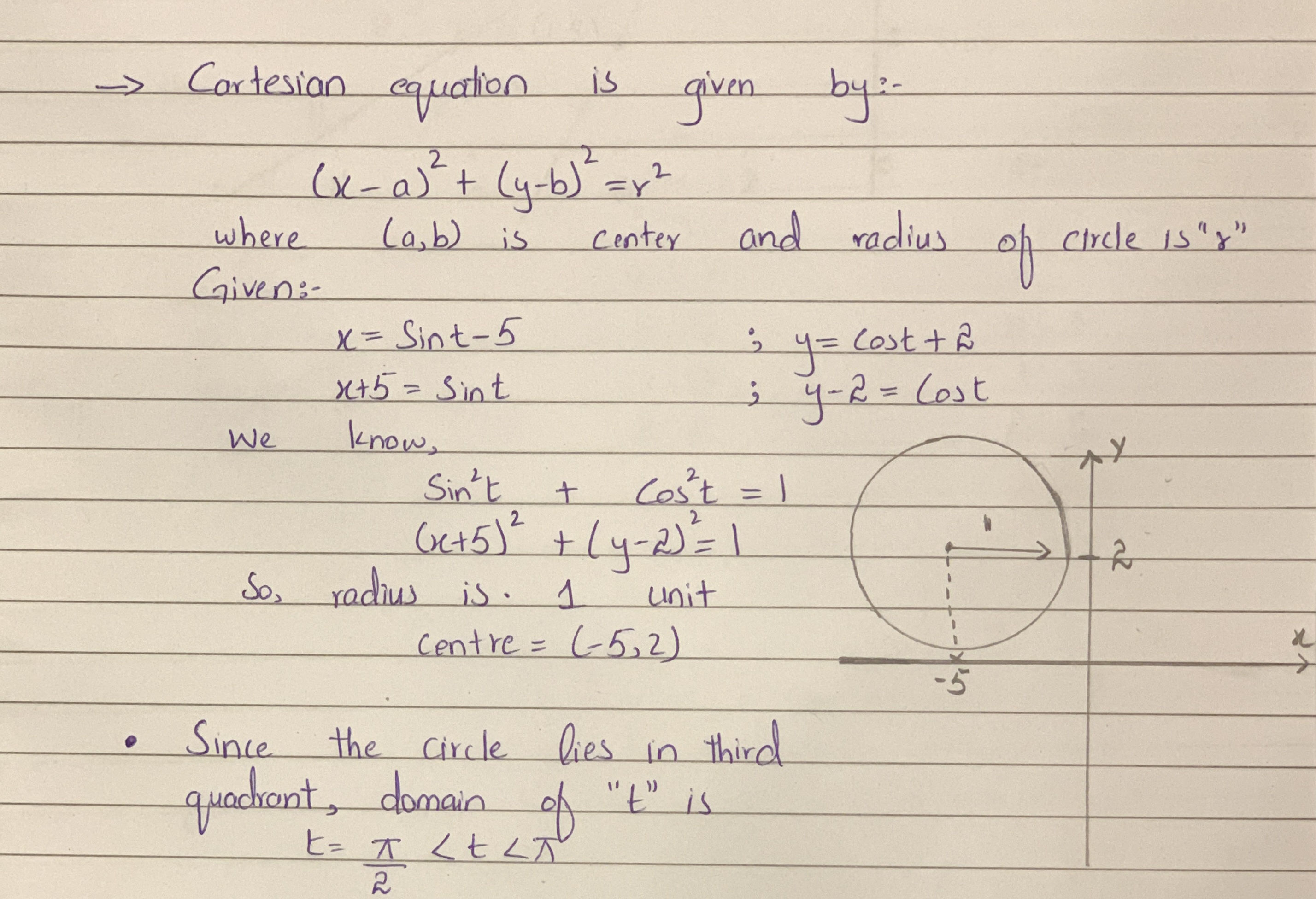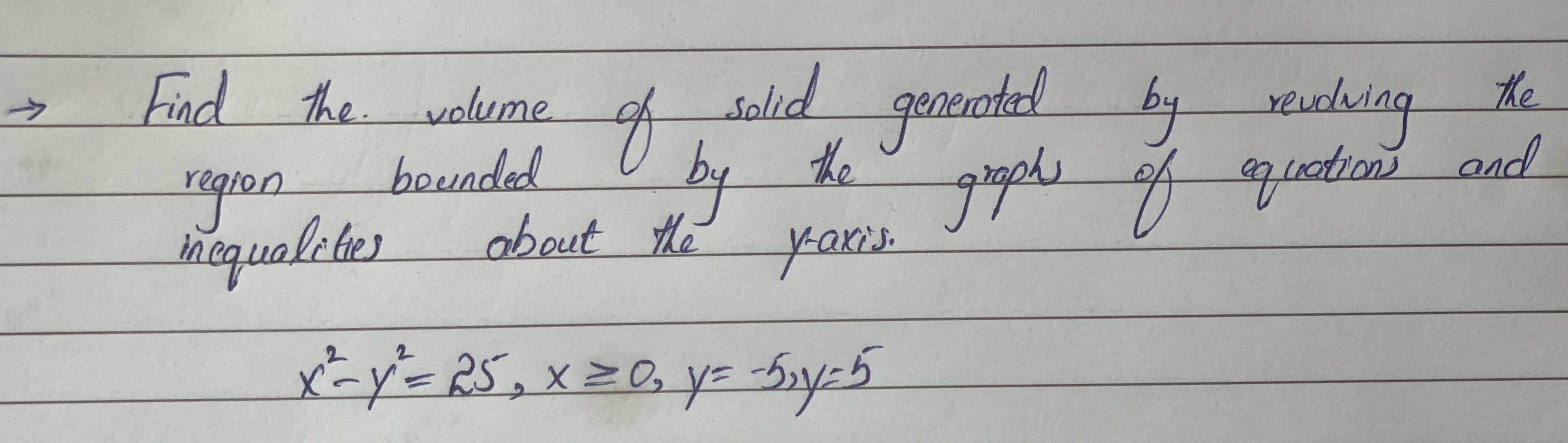# A circle has parametric equations x=sin t-5, y=cos t+2 a.Find Cartesian equation of the circle b.Write down the radius and the coordinates of the centre of the circle c. Write down a suitable domain of t which defines one full revolution around the circle. 13310200961.JPGQuestion
Equations and inequalitiesA circle has parametric equations $$\displaystyle{x}={\sin{{t}}}-{5},\ {y}={\cos{{t}}}+{2}$$
a.Find Cartesian equation of the circle
b.Write down the radius and the coordinates of the centre of the circle
c. Write down a suitable domain of t which defines one full revolution around the circle.2020-10-24### Relevant QuestionsThat parametric equations contain more information than just the shape of the curve. Write a short paragraph explaining this statement. Use the following example and your answers to parts (a) and (b) below in your explanation. The position of a particle is given by the parametric equations $$x =\ \sin\ (t)\ and\ y =\ \cos\ (t)$$ where t represents time. We know that the shape of the path of the particle is a circle. a) How long does it take the particle to go once around the circle? Find parametric equations if the particle moves twice as fast around the circle. b) Does the particle travel clockwise or counterclockwise around the circle? Find parametric equations if the particle moves in the opposite direction around the circle.Write a short paragraph explaining this statement. Use the following example and your answers Does the particle travel clockwise or anticlockwise around the circle? Find parametric equations if the particles moves in the opposite direction around the circle. The position of a particle is given by the parametric equations $$x = sin t, y = cos t$$ where 1 represents time. We know that the shape of the path of the particle is a circle.Write a short paragraph explaining this statement. Use the following example and your answers How long does it take the particle to go once around the circle? Find parametric equations if the particle moves twice as fast around the circle. The position of a particle is given by the parametric equations $$x = sin t, y = cos t$$ where 1 represents time. We know that the shape of the path of the particle is a circle.1. A curve is given by the following parametric equations. x = 20 cost, y = 10 sint. The parametric equations are used to represent the location of a car going around the racetrack. a) What is the cartesian equation that represents the race track the car is traveling on? b) What parametric equations would we use to make the car go 3 times faster on the same track? c) What parametric equations would we use to make the car go half as fast on the same track? d) What parametric equations and restrictions on t would we use to make the car go clockwise (reverse direction) and only half-way around on an interval of [0, 2?]? e) Convert the cartesian equation you found in part “a” into a polar equation? Plug it into Desmos to check your work. You must solve for “r”, so “r = ?”Eliminate the parameter and obtain the standard form of the rectangular equation. Circle: $$x = h + r cos(?), y = k + r sin(?)$$ Use your result to find a set of parametric equations for the line or conic section. $$(When 0 \leq ? \leq 2?.)$$ Circle: center: (6, 3), radius: 7Parametric to polar equations Find an equation of the following curve in polar coordinates and describe the curve. $$x = (1 + cos t) cos t, y = (1 + cos t) sin t, 0 \leq t \leq 2\pi$$Parametric equations and a value for the parameter t are given $$x = (60 cos 30^{\circ})t, y = 5 + (60 sin 30^{\circ})t - 16t2, t = 2$$. Find the coordinates of the point on the plane curve described by the parametric equations corresponding to the given value of t.Find the volume of the solid generated by revolving the revolving the region bounded by the graphs of the equations and inequalities about the y-axis
$$\displaystyle{x}^{{2}}-{y}^{{2}}={25},{x}\geq{0},{y}=-{5},{y}={5}$$Determine which of the following are linear inequalities and linear equations.Write LI if the sentence is linear inequality and LE if it is linear equation
1.2x+y>1
2.y=3x-6
3.y-5<7x
4.$$3s >= t+1$$
5.x+5y=10A skateboarding park charges $7 per session to skate and$4 per session to rent safety equipment. Jared rents safety equipment every time he skates. During one year, he spends \$99 for skating charges and equipment rentals.